• 参数回归模型在金融时间序列上的应用，朱云霓，刘琼荪，本文旨在运用非参数回归模型来解决金融上的实际问题；对1998~2009年的上证综指的收益率数据进行了简单的统计分析，说明利用非参数回
• 该方法首先基于函数型数据分析理论，将日负荷曲线视为函数型数据，通过对历史负荷曲线样本自身规律的挖掘，建立基于历史负荷曲线样本的函数型非参数回归预测模型。在此基础上，通过构建二次规划模型对函数型非参数...
• 论文研究-异方差非参数回归模型均值与方差变点的小波估计与应用.pdf, 金融市场中,受突发事件的影响反映资产平均收益的均值函数和反映资产收益波动的方差函数都有可能...
• 采用非参数方法表达不能量化的影响因素，建立了半参数回归模型，并与线性回归模型和灰色预测模型进行了比较．研究结果表明，用半参数回归模型预测铁路货物周转量，预测结果的相对误差仅1．7％，比线性回归模型和灰色...
• 在原始数据预处理的基础上，运用相关性分析理论确定了半参数模型中的参数与非参数变量，构建了半参数回归模型；利用两阶段最小二乘法和权重系数法对回归系数和回归参数进行了估计．算例结果表明，半参数回归分析法...
• 参数回归是我们最长用的模型。与参数回归相对的非参数回归，这种模型对变量分布等假定并不是很严等，因此可以说扩展了参数回归的应用范围。但是非参数回归的局限性在于，在存在较多的解释变量时，很容易出现所谓的...


参数回归是我们最长用的模型。与参数回归相对的非参数回归，这种模型对变量分布等假定并不是很严等，因此可以说扩展了参数回归的应用范围。但是非参数回归的局限性在于，在存在较多的解释变量时，很容易出现所谓的“维度灾难”，像方差的急剧增大等现象。
这类模型包括实例回归，局部加权回归（LOESS）和样条回归。非参数方法一般适用于低维空间（较少的解释变量）。该局部加权回归曲线是利用点附近的点信息，使用的点信息越多，曲线与拟合直线越接近；使用的点信息越少，与散点越吻合。在变量间非线性关联较强的情况下，相比普通回归，通常更稳健一些。
介于参数回归与非参回归之间的就是半参数模型，这种模型结合了前面两种参数模型的诸多优点，例如使用的连接函数、分析形式多样化，而且光滑参数值的确认均可以使用广义交叉验证技术。其应用情景首先是因变量在不符合正态分布时，该模型的结果仍然很稳定，我们可以选择不同的分布形式等。非参数模型的另一个典型应用是可以对具有截尾数据的资料进行生存预测。例如，普通生存分析，并没有很好的解决多解释变量的情况，并且对分布有特定的需求，而且当相关假定违反时，往往会对模型产生很大的影响，半参数生存分析回归模型克服了上述参数法的诸多局限，可以灵活地处理许多未知分布与不服从参数分布类型的资料。
另外，一个比较容易混淆的是广义可加模型（使用连接函数的可加模型），与广义线性模型很相似，主要使用非参估计的方法。【机器学习】参数和非参数机器学习算法

05-03 22:21
首页
数据科学与人工智能

什么是参数机器学习算法并且它与非参数机器学习算法有什么不同?
本文中你将了解到参数和非参数机器学习算法的区别。
让我们开始吧。
学习函数
机器学习可以总结为学习一个函数(f)，其将输入变量(X)映射为输出变量(Y)。
Y=f(x)
算法从训练数据中学习这个映射函数。
函数的形式未知，于是我们机器学习从业者的任务是评估不同的机器学习算法，然后选择好的能拟合潜在的目标函数的算法。
不同的算法对目标函数的形式和学习的方式有不同的估计和偏差。
参数机器学习算法
假设可以极大地简化学习过程，但是同样可以限制学习的内容。简化目标函数为已知形式的算法就称为参数机器学习算法。

通过固定大小的参数集(与训练样本数独立)概况数据的学习模型称为参数模型。不管你给与一个参数模型多少数据，对于其需要的参数数量都没有影响。 — Artificial Intelligence: A Modern Approach，737页

参数算法包括两部分：
选择目标函数的形式。 从训练数据中学习目标函数的系数。
对于理解目标函数来讲，最简单的就是直线了，这就是线性回归里面采用的形式:
b_0+b_1<em>x_1+b_2</em>x_2=0
其中b_0、b_1和b_2是直线的系数，其影响直线的斜度和截距，x_1和x_2是两个输入变量。
把目标函数的形式假设为直线极大地简化了学习过程。那么现在，我们需要做的是估计直线的系数并且对于这个问题预测模型。
通常来说，目标函数的形式假设是对于输入变量的线性联合，于是参数机器学习算法通常被称为“线性机器学习算法”。
那么问题是，实际的未知的目标函数可能不是线性函数。它可能接近于直线而需要一些微小的调节。或者目标函数也可能完全和直线没有关联，那么我们做的假设是错误的，我们所做的近似就会导致差劲的预测结果。
参数机器学习算法包括:
逻辑回归  线性成分分析  感知机
参数机器学习算法有如下优点:
简洁：理论容易理解和解释结果  快速：参数模型学习和训练的速度都很快  数据更少：通常不需要大量的数据，在对数据的拟合不很好时表现也不错
参数机器学习算法的局限性：
约束：以选定函数形式的方式来学习本身就限制了模型  有限的复杂度：通常只能应对简单的问题  拟合度小：实际中通常无法和潜在的目标函数吻合
非参数机器学习算法
对于目标函数形式不作过多的假设的算法称为非参数机器学习算法。通过不做假设，算法可以自由的从训练数据中学习任意形式的函数。

当你拥有许多数据而先验知识很少时，非参数学习通常很有用，此时你不需要关注于参数的选取。 — Artificial Intelligence: A Modern Approach，757页

非参数理论寻求在构造目标函数的过程中对训练数据作最好的拟合，同时维持一些泛化到未知数据的能力。同样的，它们可以拟合各自形式的函数。
对于理解非参数模型的一个好例子是k近邻算法，其目标是基于k个最相近的模式对新的数据做预测。这种理论对于目标函数的形式，除了相似模式的数目以外不作任何假设。
一些非参数机器学习算法的例子包括：
决策树，例如CART和C4.5  朴素贝叶斯  支持向量机  神经网络
非参数机器学习算法的优势：
可变性：可以拟合许多不同的函数形式。  模型强大：对于目标函数不作假设或者作微小的假设  表现良好：对于预测表现可以非常好。
非参数机器学习算法局限性：
需要更多数据：对于拟合目标函数需要更多的训练数据  速度慢：因为需要训练更多的参数，训练过程通常比较慢。  过拟合：有更高的风险发生过拟合，对于预测也比较难以解释。
延伸阅读
对于参数和非参数机器学习算法的不同以下是一些资源。
书籍
An Introduction to Statistical Learning: with Applications in R，章节2 Artificial Intelligence: A Modern Approach，章节18
网页
机器学习中使用非参数理论的好处是什么？ Quora 机器学习中使用非参数理论的缺点是什么？ Quora 非参数统计 维基百科 参数统计维基百科 参数vs非参数 StackExchange
总结
本文中你了解到了参数和非参数机器学习算法的不同之处。
你学习到，参数理论对于映射函数做很多的假设，这使得模型易于训练，需要的数据量少，同时也使得模型能力有限。
非参数理论对于目标函数的形式不作过多的假设，这使得模型需要更多的数据来训练，并且模型拥有高复杂度，同时也使得模型能力很强。
关于参数和非参数机器学习算法，你有什么问题吗？欢迎留下评论，我将竭力解答。
关于偏差、方差和偏差-方差的权衡，你有什么问题吗？欢迎留下评论，我将竭力解答。
原文链接：[Parametric and Nonparametric Machine Learning Algorithms(http://machinelearningmastery.com/parametric-and-nonparametric-machine-learning-algorithms/ “Parametric and Nonparametric Machine Learning Algorithms”)

﻿﻿

展开全文• 基于上述目的，本文从最小二乘配置的理论和模型出发，在分析、研究近年来关于统计分析和测量数据处理有关文献的基础上，引入半参数回归分析的概念，并讨论了正则化参数α对解的影响。通过模拟计算得出结论：只要正则
• 基于稳健M-估计所得到的散布矩阵，给出了半参数回归模型中回归参数β的稳健估计，并证明了估计量的强相合性以及渐近正态性。
• 参数回归模型(包括部分线性回归模型)是当今统计学界研究的热点课题之一，研究了一类基于网点观测的部分线性回归模型，利用最小二乘法给出了未知参数和误差方差的估计，在一般条件下，证明了估计量的渐近正态性。
• 构造了基于小波系数的多变点检测算法,该算法可应用于非参数回归模型多变点检测.渚河水文时间序列实例分析结果表明,检测的结果与实际情况相符合.该方法不需要对时间序列做任何参数化的假定便能方便地检测出变点的位置...
• 本文考虑一类固定设计的半参数回归模型，其误差为一阶自回归时间序列。用权函数及拟极大似然估计方法得到了一些参数及非参数的拟极大似然估计量，在适当的条件下，研究了它们的弱相合性，从而丰富了该类半参数回归...
• 运用Ratio检验方法研究相依误差下非参数回归模型的方差变点检验.首先利用核估计方法估计模型中的回归函数得到残差序列，其次利用残差序列构造Ratio检验统计量，并推导检验统计量的极限分布，最后通过数值模拟验证...
• 研究了一类纵向污染数据的半参数回归模型，并在更切合实际的情况下，建立了模型参数和回归函数的估计方法．在适当的条件下，证明了所建立的估计量均具有强相合性．
• 在贝叶斯框架下,研究了一种特殊的非参数回归模型的最优设计问题,给出了贝叶斯非参数回归设计的准则,并在这一准则下证明了均匀设计在该模型下具有允许性和极小极大性。
• NA样本下一类半参数回归模型的强相合性，张燕，赵选民，考虑固定设计下一类半参数回归模型 ，且随机误差 为NA序列且。利用最小二乘估计和非参数函数的权函数估计的方法，给出了参数的估�
• 论文研究-基于小波的非参数回归模型均值变点的Bootstrap监测.pdf, 本文首次考虑非参数回归模型均值函数结构变点的在线监测问题. 首先对回归函数的局部线性估计值进行...
• 1、回归分析概括 目标值（因变量）是连续型数据，通过某种函数关系找到因变量和自变量之间的关系，进而预测目标。 常见的回归：线性回归、岭回归、非线性回归 回归拟合目标：计算自变量与因变量关系的函数参数 通过...
• 最后,提出油气勘探中预测孔隙度的问题,并用逐步回归分析优化回归方程并用此方程预测岩芯属性参数。研究表明,该方法预测精度高,方法稳定有效,逐步回归较好的解决了部分测井勘探的实际问题,基于多种测井信息的多元线性...
• 特别是，五参数对数回归或5PL非线性回归模型通常用于生物测定或免疫测定（例如ELISA，RIA，IRMA或剂量React曲线）中的曲线拟合分析。 标准剂量React曲线有时称为五参数逻辑方程。 它的特点是其经典的“S”或 S 形...matlab
• ## 回归分析参数介绍

千次阅读 2020-01-10 18:07:20
回归误差平方和（SSE） 残差平方和（SSR）及总体平方和（SST） df（degree of freedom）为自由度。 MS为SS与df的比值，与SS对应，SS是平方和，MS是均方，是指单位自由度的平方和。 coeft表明系数的，因为该因素t...
SS是平方和
回归误差平方和（SSE）
残差平方和（SSR）及总体平方和（SST）
df（degree of freedom）为自由度。
MS为SS与df的比值，与SS对应，SS是平方和，MS是均方，是指单位自由度的平方和。
coeft表明系数的，因为该因素t检验的P值是0.000，所以表明有很强的正效应，认为所检验的变量对模型是有显著影响的。
F是F test F 检验，联合显著检验值，是表明相关性的系数
展开全文• ## 非参数回归

千次阅读 多人点赞 2020-04-21 21:14:51
一元非参数回归 给定一组样本观测值(Y1,X1),(Y2,X2),⋯ ,(Yn,Xn),(Y_1,X_1),(Y_2,X_2),\cdots,(Y_n,X_n),(Y1​,X1​),(Y2​,X2​),⋯,(Yn​,Xn​),XiX_iXi​和YiY_iYi​之间的任意函数模型表示为 Yi=m(Xi)+εi,i=1,...
一元非参数回归
给定一组样本观测值

(

Y

1

,

X

1

)

,

(

Y

2

,

X

2

)

,

⋯

,

(

Y

n

,

X

n

)

,

(Y_1,X_1),(Y_2,X_2),\cdots,(Y_n,X_n),

X

i

X_i

和

Y

i

Y_i

之间的任意函数模型表示为

Y

i

=

m

(

X

i

)

+

ε

i

,

i

=

1

,

2

,

⋯

,

n

.

Y_i=m(X_i)+\varepsilon_i,i=1,2,\cdots,n.

其中

m

(

⋅

)

=

E

(

Y

∣

X

)

,

ε

m(\cdot)=E(Y|X),\varepsilon

为随机误差项,一般假定

E

(

ε

∣

X

=

x

)

=

0

E(\varepsilon|X=x)=0

,

v

a

r

(

ε

∣

X

=

x

)

=

σ

2

\rm{var}(\varepsilon|X=x)=\sigma^2

,不必是常数。
核回归光滑模型
参考上篇文章讲到的核密度估计法,相当于求

x

x

附件的平均点数,平均点数的求法是对可能影响到

x

x

的样本点,按照距离

x

x

的远近作距离加权平均.核回归光滑的基本思路之类似，在此不是求平均点数,而是估计点

x

x

处

y

y

的取值,仍然按照距离

x

x

的远近对样本观测值

y

i

y_i

加权,这一思想被称为

N

a

d

a

r

a

y

a

−

W

a

t

s

o

n

核回归.
选择核函数

K

(

⋅

)

K(\cdot)

以及窗宽

h

n

>

0

h_n>0

,

inf

⁡

K

(

u

)

d

u

=

1.

\inf K(u)\rm{d}u=1.

定义加权平均核为

ω

i

(

x

)

=

K

h

n

(

X

i

−

x

)

∑

j

=

1

n

K

h

n

(

X

j

−

x

)

,

i

=

1

,

2

,

⋯

,

n

,

\omega_i(x)=\frac{K_{h_n}(X_i-x)}{\sum_{j=1}^n K_{h_n}(X_j-x)}, i=1,2,\cdots,n,

其中

K

h

n

(

u

)

=

h

n

−

1

K

(

u

h

n

−

1

)

K_{h_n}(u)=h_n^{-1}K(uh_n^{-1})

是一个概率密度函数.则N-W估计的定义为:

m

n

(

x

)

=

∑

i

=

1

n

^

ω

i

(

x

)

Y

i

.

\hat{m_n(x)=\sum_{i=1}^n}\omega_i(x)Y_i.

注意一点：

θ

^

=

min

⁡

θ

∑

i

=

1

n

ω

i

(

x

)

(

Y

i

−

θ

)

2

=

∑

i

=

1

n

ω

i

Y

i

∑

i

=

1

n

ω

i

,

\hat{\theta}=\min_\theta \sum_{i=1}^n \omega_i(x)(Y_i-\theta)^2=\sum_{i=1}^n \frac{\omega_iY_i}{\sum_{i=1}^n\omega_i},

因此,核估计等价于局部加权最小二乘估计.权重

ω

i

=

K

(

X

i

−

x

)

\omega_i=K(X_i-x)

.常用核函数已经在上篇文章介绍.核密度回归还有另外一种写法:

E

(

Y

∣

X

=

x

)

=

∫

y

f

(

y

∣

x

)

d

y

=

∫

y

f

(

x

,

y

)

f

X

(

x

)

d

y

=

m

(

x

)

,

E(Y|X=x)=\int yf(y|x)dy=\int y \frac{f(x,y)}{f_X(x)}dy=m(x),

其中

f

(

y

∣

x

)

f(y|x)

是给定

X

=

x

X=x

时

Y

Y

的条件pdf,

f

X

(

x

)

f_X(x)

是

X

X

的边际pdf.上式对

y

y

积分所以可以进一步写为:

m

(

x

)

=

E

(

Y

∣

X

=

x

)

=

∫

y

f

(

x

,

y

)

d

y

f

X

(

x

)

.

m(x)=E(Y|X=x)=\frac{\int y f(x,y)dy}{f_X(x)}.

给定样本观测值为

{

X

i

,

Y

i

}

,

i

=

1

,

…

,

n

\{X_i,Y_i\},i=1,\dots,n

.此时的未知量是

f

(

x

,

y

)

f(x,y)

和

f

X

(

x

)

f_X(x)

,我们可以通过多元核密度估计来得到,此时有:

f

^

h

,

g

(

x

,

y

)

=

1

n

∑

i

=

1

n

K

h

(

x

−

X

i

h

)

K

g

(

y

−

Y

i

g

)

\hat{f}_{h,g}(x,y)=\frac{1}{n}\sum_{i=1}^n K_h \left( \frac{x-X_i}{h} \right)K_g \left( \frac{y-Y_i}{g} \right)

令

(

y

−

Y

i

)

g

=

s

\frac{(y-Y_i)}{g}=s

:

∫

y

f

^

h

,

g

(

x

,

y

)

d

y

=

1

n

∑

i

=

1

n

1

h

K

(

x

−

X

i

h

)

∫

y

g

K

g

(

y

−

Y

i

g

)

d

y

=

1

n

∑

i

=

1

n

K

h

(

x

−

X

i

)

∫

(

s

g

+

Y

i

)

K

(

s

)

d

s

=

1

n

K

h

(

x

−

X

i

)

{

g

∫

s

K

(

s

)

d

s

+

Y

i

∫

K

(

s

)

d

s

}

=

1

n

K

h

(

x

−

X

i

)

Y

i

\begin{aligned} \int y\hat{f}_{h,g}(x,y)dy &=\frac{1}{n}\sum_{i=1}^n \frac{1}{h}K\left( \frac{x-X_i}{h} \right)\int\frac{y}{g}K_g\left( \frac{y-Y_i}{g} \right)dy\\ &=\frac{1}{n}\sum_{i=1}^nK_h(x-X_i)\int(sg+Y_i)K(s)ds\\ &= \frac{1}{n}K_h(x-X_i) \left\{g\int sK(s)ds+Y_i\int K(s)ds \right\} \\ &=\frac{1}{n}K_h(x-X_i)Y_i \end{aligned}

其中由核函数的性质得到:

∫

s

K

(

s

)

d

s

=

E

(

s

)

=

0

\int sK(s)ds=E(s)=0

,

∫

K

(

s

)

d

s

=

1

\int K(s)ds=1

m

^

h

(

x

)

=

n

−

1

∑

i

=

1

n

K

h

(

x

−

X

i

)

Y

i

n

−

1

∑

j

=

1

n

K

h

(

x

−

X

j

)

\hat{m}_h(x)=\frac{n^{-1}\sum_{i=1}^nK_h(x-X_i)Y_i}{n^{-1}\sum_{j=1}^{n}K_h(x-X_j)}

举个例子：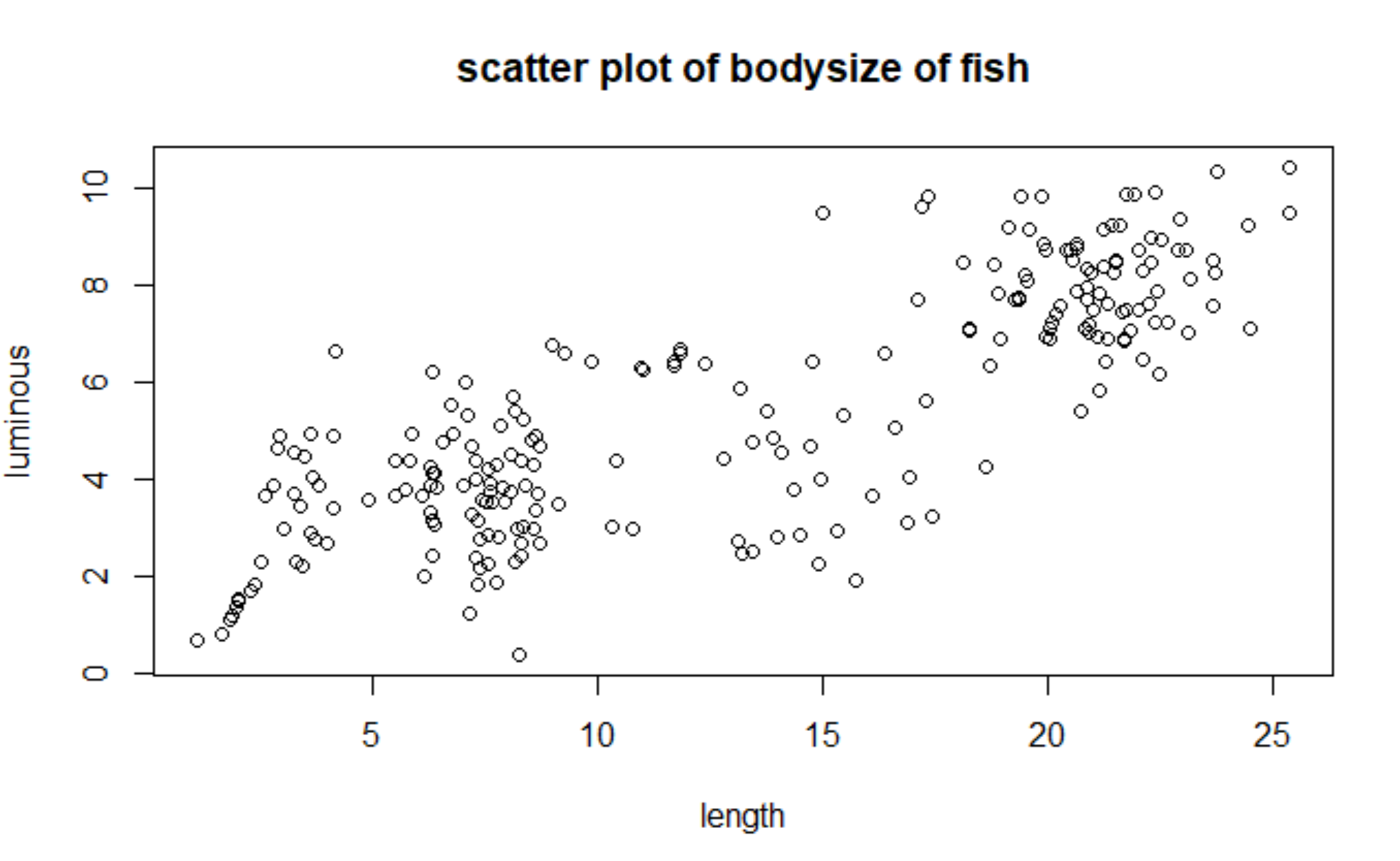我们通过一元核回归模型来拟合这个数据集,采用

N

a

d

a

r

a

y

a

−

W

a

t

s

o

n

核回归,同时比较不同窗宽

h

h

对回归曲线的影响,首先复习一下上节的二元核密度估计: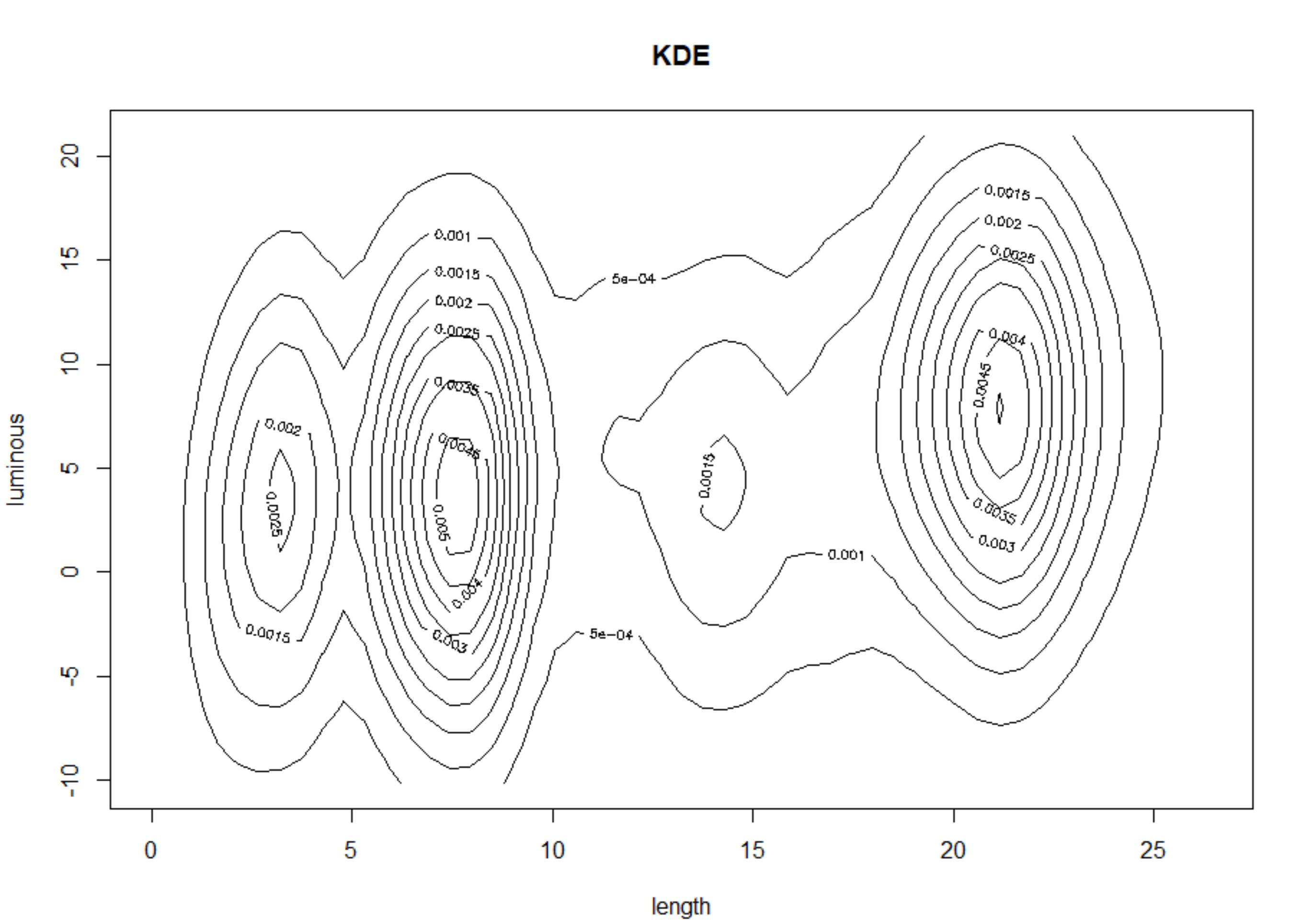再看看另一个角度：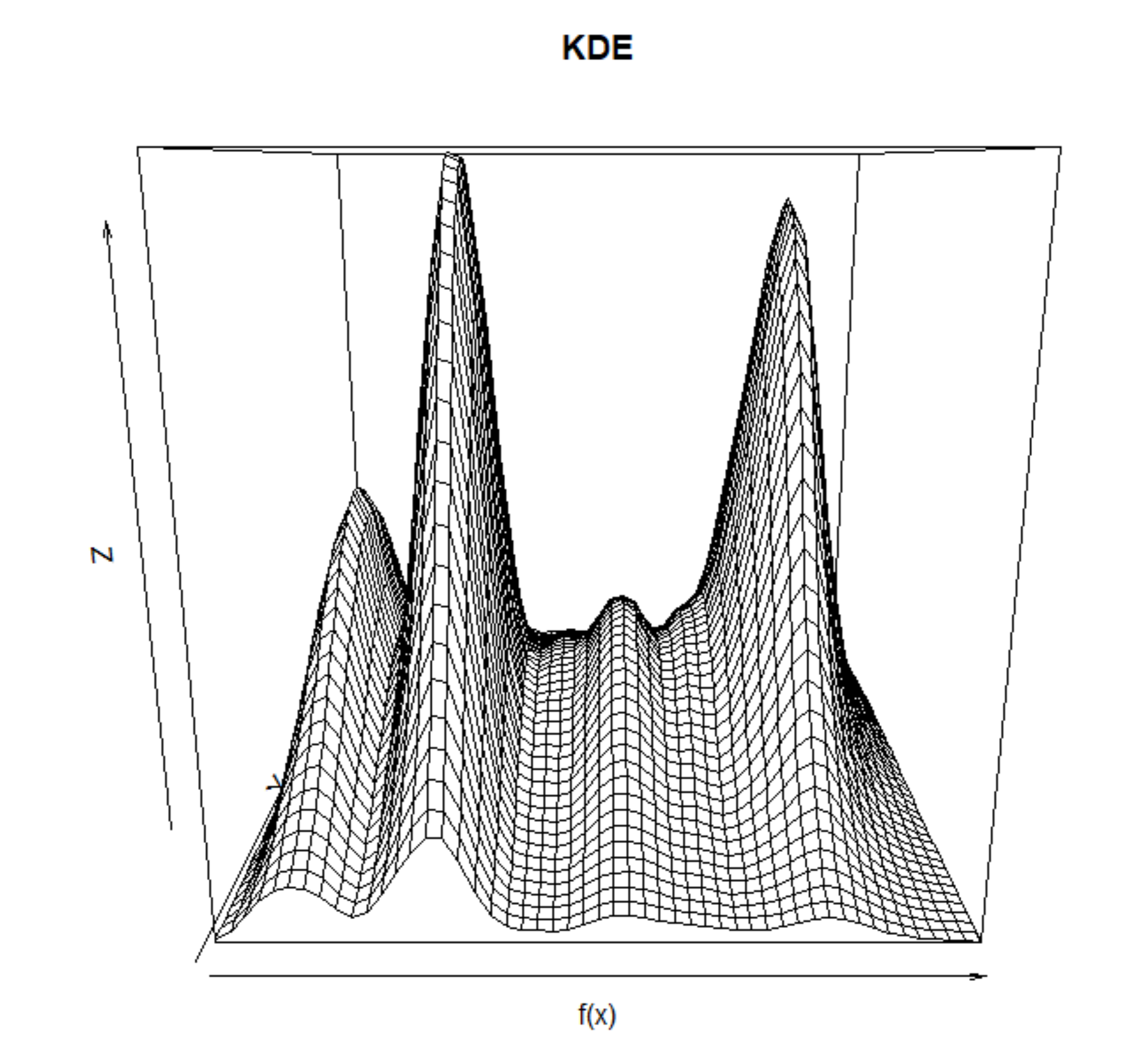代码如下
file = "D:\REF\fish.txt"
x <- cbind(data$length,data$luminous)

est <- bkde2D(x=x)
contour(est$x1, est$x2, est$fhat,xlab = 'length',ylab='luminous',main='KDE') persp(est$fhat,xlab = 'f(x)',main = 'KDE')

再进行核回归,这里分别取

h

=

0.1

,

0.5

,

1.5

,

3.0

h=0.1,0.5,1.5,3.0

.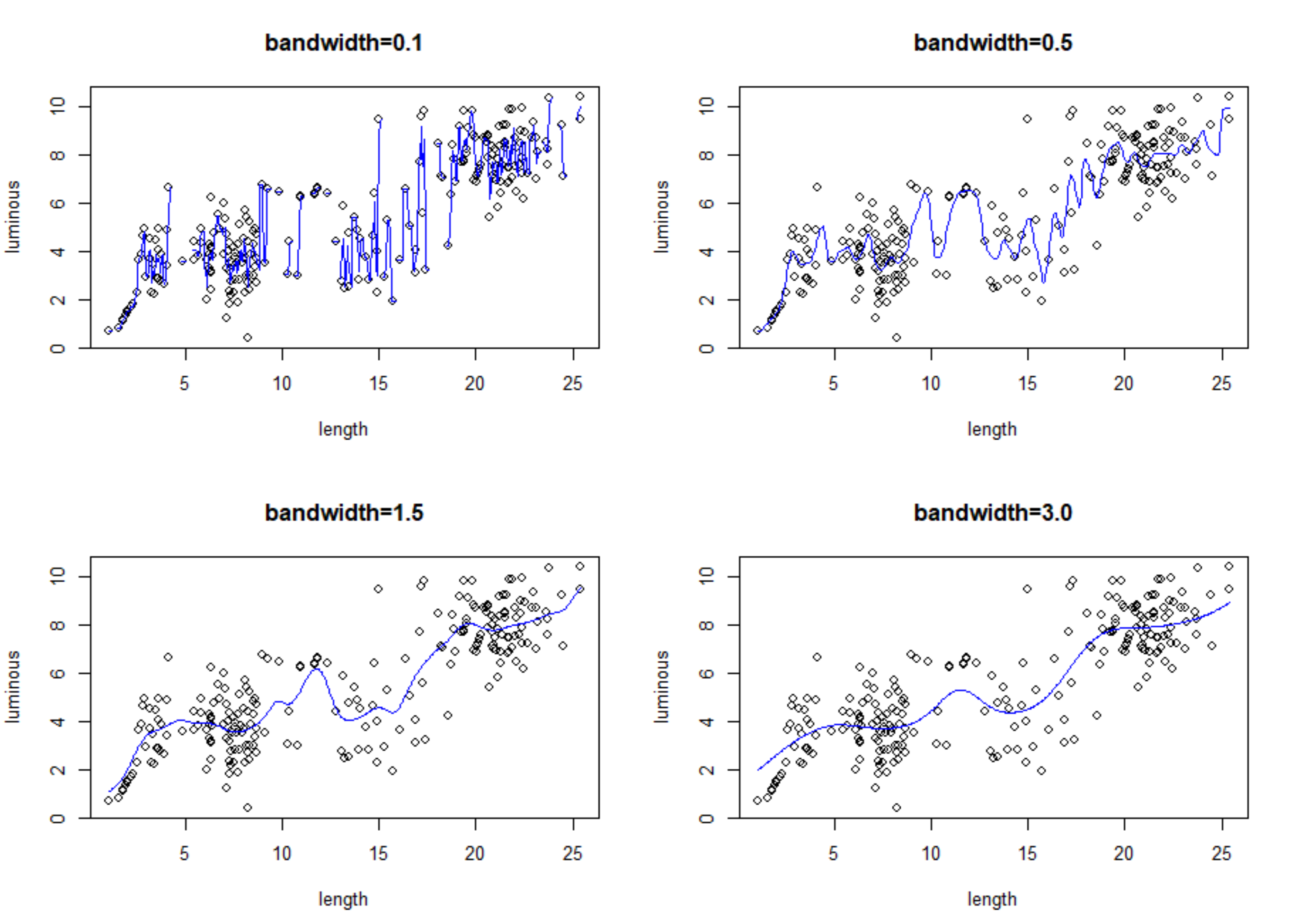R语言代码如下
file = "D:\REF\fish.txt"
x <- cbind(data$length,data$luminous)

par(mfrow=c(2,2))
fit1 <- ksmooth(x=data$length,y=data$luminous,kernel='normal',bandwidth = 0.1,range.x = range(data$length),n.points = length(x)) fit2 <- ksmooth(x=data$length,y=data$luminous,kernel='normal',bandwidth = 0.5,range.x = range(data$length),n.points = length(x))
fit3 <- ksmooth(x=data$length,y=data$luminous,kernel='normal',bandwidth = 1.5,range.x = range(data$length),n.points = length(x)) fit4 <- ksmooth(x=data$length,y=data$luminous,kernel='normal',bandwidth = 3.0,range.x = range(data$length),n.points = length(x))
plot(x1,x2,xlab = 'length' ,ylab = 'luminous' ,main='bandwidth=0.1')
lines(fit1,lwd=1.0,col='blue')
plot(x1,x2,xlab = 'length' ,ylab = 'luminous' ,main='bandwidth=0.5')
lines(fit2,lwd=1.0,col='blue')
plot(x1,x2,xlab = 'length' ,ylab = 'luminous' ,main='bandwidth=1.5')
lines(fit3,lwd=1.0,col='blue')
plot(x1,x2,xlab = 'length' ,ylab = 'luminous' ,main='bandwidth=3.0')
lines(fit4,lwd=1.0,col='blue')

展开全文• 回归分析中要确定一个一元线性回归方程，很简单，只需要通过样本数据求出公式中a和b两个参数的值，一元线性回归方程就唯一确定。 a和b如何确定，有一种比较好的计算方法，叫做最小二乘法。这里以一个简单小
一、一元线性回归
回归分析可以用来分析变量间的关联强度和关联方向，而且还可以通过回归方程式，利用已知的自变量预测未知的因变量。
一元线性回归又称简单线性回归，是只包括一个自变量和一个因变量，而且二者的关系可以用一条直线近似表示，会建立出一个一元线性回归方程。设为二、最小二乘法求一元线性回归方程
回归分析中要确定一个一元线性回归方程，很简单，只需要通过样本数据求出公式中a和b两个参数的值，一元线性回归方程就唯一确定。

a和b如何确定，有一种比较好的计算方法，叫做最小二乘法。这里以一个简单小案例具体介绍如何用最小二乘法估计参数a和b。

案例数据：为了研究受教育年限和职业声望之间的关系，得到了8个抽样调查结果如下：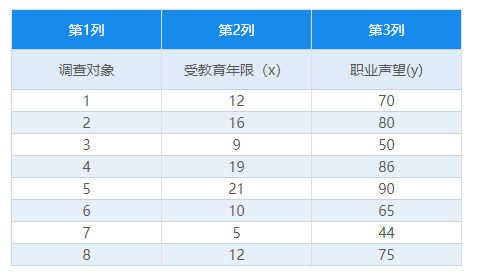首先分析样本数据，先绘制一个散点图，看看数据的分布情况，这里将数据整理成上方表格的形式，上传到SPSSAU，上传成功如下图所示，【受教育年限】为自变量，【职业声望】为因变量：选择【可视化】板块中的【散点图】，以受教育年限】和【职业声望】两个变量的数据来绘制散点图：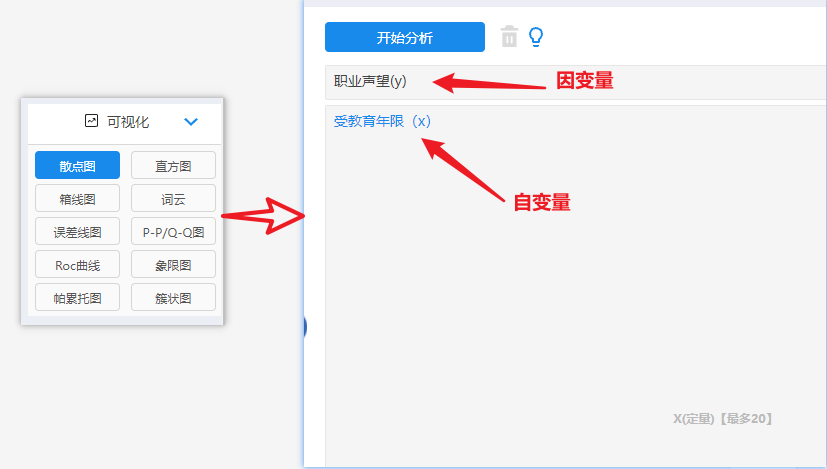输出散点图：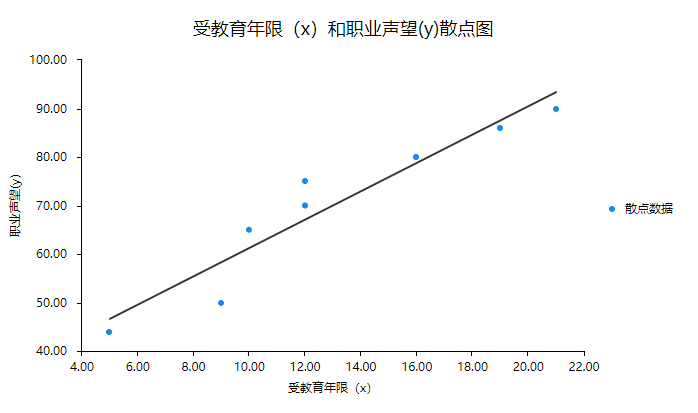由散点图可见，职业声望（Y）随受教育年限（X）的增加而增加，且这些点呈直线趋势，所以我们可以求出一条最能代表这8个数据分布点分布趋势的直线：
不妨设为：使用最小二乘法对这个直线回归方程中的参数a和b进行估计:
公式如下：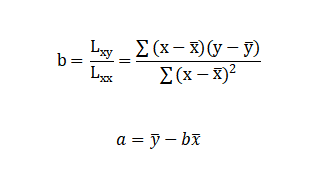具体求法：
第一步：求出变量x的平均值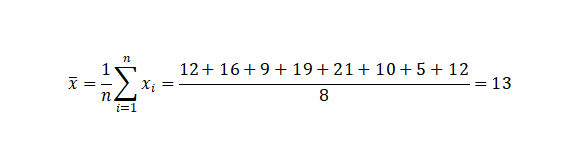第二步：求出变量y的平均值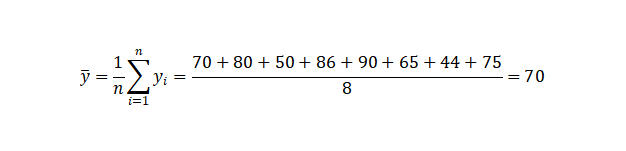第三步：求出系数b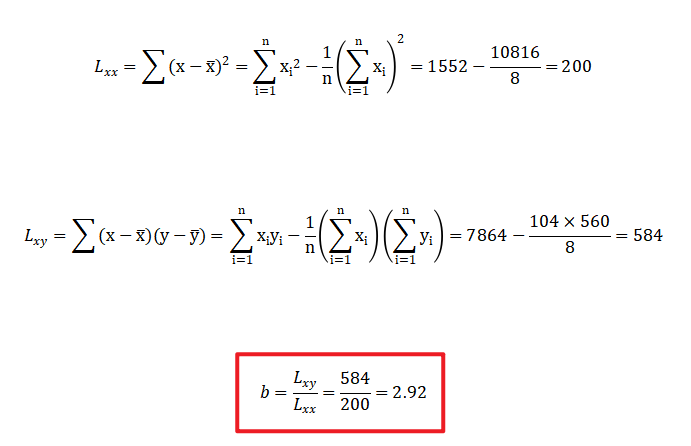第四步：求出截距a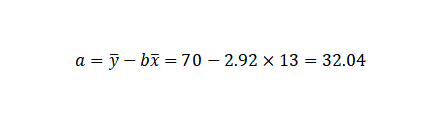这样就得到了一元线性回归方程：三、SPSSAU线性回归分析
需要对数据进行线性回归分析，可以使用SPSSAU，快速完成数据分析，在【通用方法】中选中【线性回归】方法，将【受教育年限】与【职业声望】分别放入对应分析框中，点击【开始分析】可一键输出结果，如下图：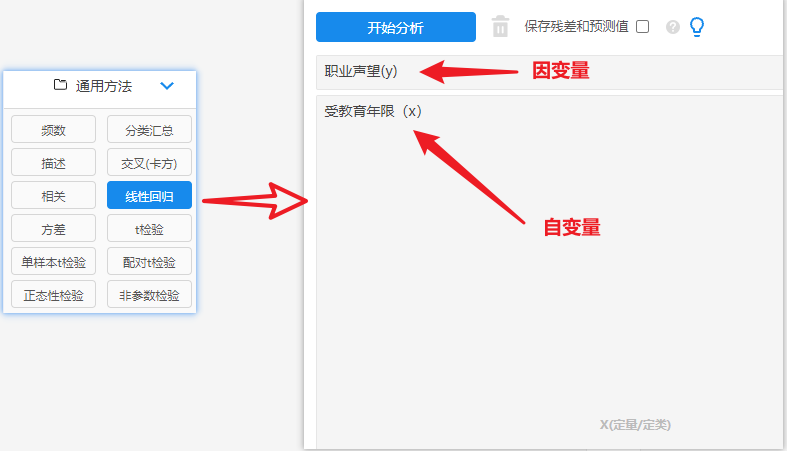输出结果：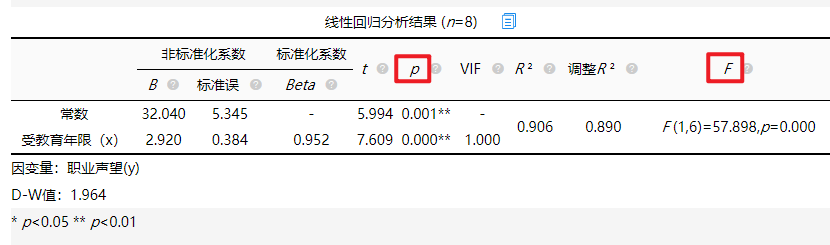智能分析：由文字分析可知，SPSSAU算出的线性回归模型公式和我们之前手算的公式完全一样，另外自动分析出受教育年限会对职业声望产生显著的正向影响关系。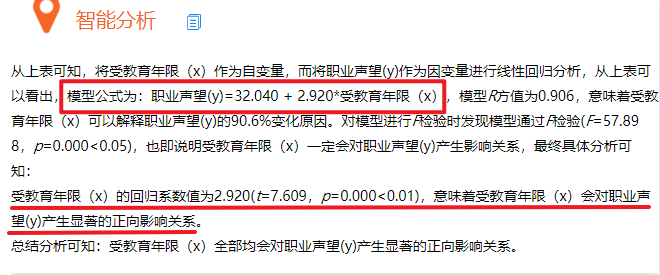模型预测：另外还提供简单线性回归的模型预测，输入自变量【受教育年限】的值，系统会自动预测【职业声望】的值。如下图：此时我们预测受教育年限为25时的职业声望，如下图：另外可以通过线性回归的方差分析检验回归模型：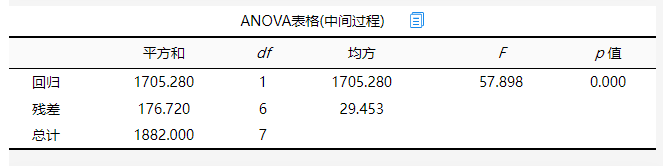方差分析可知，对模型进行F检验时发现模型通过F检验(F=57.898，p=0.000<0.05)，也即说明模型构建有意义。
展开全文• 回归分析： x变化一单位时，y平均变化多少 如果我们能够确定这条直线，我们就可以用直线的斜率来表示当x变化一单位时y的变化程度，由图中的点确定线的过程就是回归。 β1、β2为参数（parameters）,或称...
• 利用经验似然方法研究一元线性回归模型参数的检验问题，构造了相关检验的检验统 计量，并在零假设下获得了检验统计量的极限分布.结果表明，该方法可用于多克隆抗体稀 释倍数与有色物吸光度值（O D值）的建模.
• matlab多元参数非线性回归模型代码NEU 337-2020年Spring（53760） 现代神经科学的程序设计和数据分析 !!! 请在第一类之前安装Python和JupyterLab （请参阅下面的说明）。 如果遇到任何麻烦，请不要踩踏。 首先，在...
• 研究广义随机系数自回归模型参数的估计问题,给出了未知参数的一个估计类,证明了该估计类中估计的相合性和渐近正态性,并且获得了该估计类中的最小渐近方差估计,并通过数值模拟比较了估计类中各种估计方法的优劣.
• 本文研究基于验证数据的非参数回归测量误差模型的估计方法。 估计程序基于正交序列估计和截断序列逼近方法，没有指定任何结构方程式和分布假设。 推导了所提出的估计量的收敛速度。 通过示例和仿真，该方法对测量......